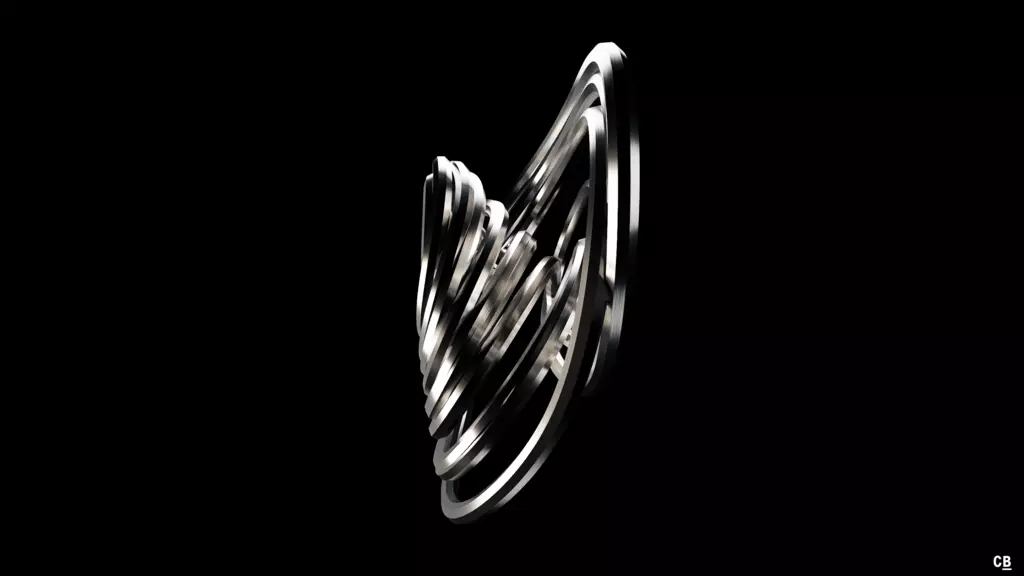# Arnéodo Attractor

In 1979 and a follow-up work in 1981, the physicist Alain Arnéodo who researches on fields like chemical chaos and fractal growth defined together with two colleagues a new type of chaotic systems1,2. This was done through a geometrical proof of Shil’nikov’s theorem which constrains a specific form of differential system so that their orbits become unstable.

The original set was:

$\dot{x} = \varrho\,x - \omega\,y + P(x,y,z)$ $\dot{y} = \omega\,x + \varrho\,y + Q(x,y,z)$ $\dot{z} = \lambda\,z + R(x,y,z)$

Where $$\lambda > -\varrho > 0$$.

## Renders

Differential system:

$\dot{x} = y$ $\dot{y} = z$ $\dot{z} = \alpha\,z - \beta\,x - \gamma\,y - x^3$

Constants:

$\alpha = -1$ $\beta = -5.5$ $\gamma = 3.5$With different constants:

$\alpha = -0.45$ $\beta = -0.8$ $\gamma = 1.1$1. P. Coullet, C. Tresser and A. Arneodo , 1979. "Transition to stochasticity for a class of forced oscillators". Phys. Lett. 72(4-5). doi:10.1016/0375-9601(79)90464-X

2. A. Arneodo, P. Coullet and C. Tresser , 1981. "Possible New Strange Attractors With Spiral Structure". Commun. Math. Phys. 79. doi:10.1007/BF01209312

🕸 💍